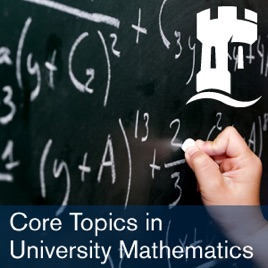20 episodes

This series of videos is aimed primarily at first-year undergraduate mathematics students.
It covers some of the topics which students often find hard, but which are fundamental to success in university mathematics.
One example of such a topic is curve sketching, which can reveal the qualitative behaviour of a function where perhaps the mathematical expression for the function is rather impenetrable. Proficiency with curve sketching comes with practice, and it is often hard to know where to begin. The videos in this series provide some useful tips.
Another example of a core topic in university mathematics is the idea of proof. Rigorous proof allows us to establish the truth of mathematical statements. Videos in this series highlight two ways of proving mathematical statements: by induction and by contradiction. Further videos highlight the generalisation of a familiar pre-university mathematical concept (a vector) to a more abstract setting (vector spaces).# Core Topics in University Mathematics University of Nottingham

• Courses

This series of videos is aimed primarily at first-year undergraduate mathematics students.
It covers some of the topics which students often find hard, but which are fundamental to success in university mathematics.
One example of such a topic is curve sketching, which can reveal the qualitative behaviour of a function where perhaps the mathematical expression for the function is rather impenetrable. Proficiency with curve sketching comes with practice, and it is often hard to know where to begin. The videos in this series provide some useful tips.
Another example of a core topic in university mathematics is the idea of proof. Rigorous proof allows us to establish the truth of mathematical statements. Videos in this series highlight two ways of proving mathematical statements: by induction and by contradiction. Further videos highlight the generalisation of a familiar pre-university mathematical concept (a vector) to a more abstract setting (vector spaces).

• video
01. Proof by Induction

## 01. Proof by Induction

This video describes how Proof by Induction works and applies it to an example.

• 7 min
• video
10. Proof by Induction

## 10. Proof by Induction

This video describes the induction step in Proof by Induction.

• 9 min
• video
09. Exponential Function

## 09. Exponential Function

This video tells us some things we never knew about the exponential function!

• 7 min
• video
05. Bases for Vector Spaces

## 05. Bases for Vector Spaces

This video describes the concept of a basis for a vector space.

• 9 min
• video
04. Intro to Vector Spaces

## 04. Intro to Vector Spaces

This video shows the ABC of Vector Spaces, with examples.

• 9 min
• video
08. Think of a Function

## 08. Think of a Function

This video discusses how to choose a function with specified properties.

• 13 min